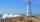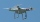# Angle + cotangens - math problems

#### Number of problems found: 22

• AngleA straight line p given by the equation ?. Calculate the size of angle in degrees between line p and y-axis.
• BuildingHow high is the building that throws horizontal shadow 95.4 m long at angle 50°?
• AircraftThe plane flies at altitude 6500 m. At the time of first measurement was to see the elevation angle of 21° and second measurement of the elevation angle of 46°. Calculate the distance the plane flew between the two measurements.
• Depth angleFrom a cliff of 150 meters high, we can see the ship at a depth angle of 9° at sea. How far is the ship from the cliff?
• TreeBetween points A and B is 50m. From A we see a tree at an angle 18°. From point B we see the tree in three times bigger angle. How tall is a tree?
• Traffic signThere is a traffic sign for climbing on the road with an angle of 7%. Calculate at what angle the road rises (falls).
• How farFrom the top of a lighthouse 145 ft above sea level, the angle of depression of a boat 29°. How far is the boat from the lighthouse?
• TV towerCalculate the height of the television tower if an observer standing 430 m from the base of the tower sees the peak at an altitude angle of 23°?
• Balloon and bridgeFrom the balloon, which is 92 m above the bridge, one end of the bridge is seen at a depth angle of 37° and the second end at depth angle 30° 30 '. Calculate the length of the bridge.
• Powerplant chimneyFrom the window of the building at a height of 7.5 m, the top of the factory chimney can be seen at an altitude angle of 76° 30 ′. The base of the chimney can be seen from the same place at a depth angle of 5° 50 ′. How tall is the chimney?
• FighterA military fighter flies at an altitude of 10 km. From the ground position, it was aimed at an altitude angle of 23° and 12 seconds later at an altitude angle of 27°. Calculate the speed of the fighter in km/h.
• The rescue helicopterThe rescue helicopter is above the landing site at a height of 180m. The site of the rescue operation can be seen from here at a depth angle of 52° 40 '. How far will the helicopter land from the rescue site?
• Tetrahedral pyramidDetermine the surface of a regular tetrahedral pyramid when its volume is V = 120 and the angle of the sidewall with the base plane is α = 42° 30´.
• A droneA flying drone aimed the area for an architect. He took off perpendicularly from point C to point D. He was at a height of 300 m above the plane of ABC. The drone from point D pointed at a BDC angle of 43°. Calculate the distance between points C and B in
• Observation towerFrom the observation tower at a height of 105 m above sea level, the ship is aimed at a depth angle of 1° 49´. How far is the ship from the base of the tower?
• KLM triangleFind the length of the sides of the triangle KLM if m = 5cm height to m = 4.5 cm and size MKL angle is 70 degrees.The ladder touch on a wall at a height of 7.5 m. The angle of the inclination of the ladder is 76°. How far is the lower end of the ladder from the wall?
• Tetrahedral pyramid 8Let’s all side edges of the tetrahedral pyramid ABCDV be equally long and its base let’s be a rectangle. Determine its volume if you know the deviations A=40° B=70° of the planes of adjacent sidewalls and the plane of the base and the height h=16 of the p
• Depth anglesAt the top of the mountain stands a castle, which has a tower 30 meters high. We see the crossroad in the valley from the top of the tower and heel at depth angles of 32° 50 'and 30° 10'. How high is the top of the mountain above the crossroad
• Cone sideCalculate the volume and area of the cone whose height is 10 cm and the axial section of the cone has an angle of 30 degrees between height and the cone side.

Do you have an interesting mathematical word problem that you can't solve it? Submit a math problem, and we can try to solve it.

We will send a solution to your e-mail address. Solved examples are also published here. Please enter the e-mail correctly and check whether you don't have a full mailbox.

Please do not submit problems from current active competitions such as Mathematical Olympiad, correspondence seminars etc...

Angle Problems. Cotangens - math word problems.